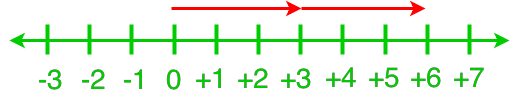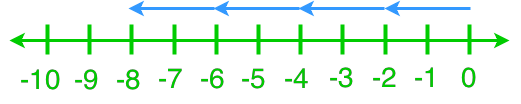# Multiplying integers

### Multiplying integers

Continue from the previous section, we will practice more on multiplication of positive and negative integers. We will look at questions involving multiplying integers and number lines. In addition, we will learn how to estimate double digit integer multiplication.

#### Lessons

• Any number multiplied by 0 will equal to 0
• 1.
Understanding Multiplication Using a Number Line
Write the multiplication statement for each number line.
a)b)• 2.
Multiplication of 1-digit Integers
Use a number line to find the product.
a)
(+4)×(+4)

b)
(+3)×(-8)

c)
(-6)×(-2)

• 3.
Multiplication of 2-digit Integers
Estimate. Then calculate.
a)
(+18)×(-33)

b)
(-21)×(+46)

c)
(-37)×(-19)

• 4.
Multiplication of Integers With Different Digits
Solve the following expressions.
a)
(+36)×(-5)

b)
(-86)×0

c)
(+167)×(+54)

d)
(-245)×(-119)

• 5.
Leo has to read 15 books during the school year. If each book has 334 pages, how many pages in total does Leo have to read?